Related Articles

# Red-Black Tree | Set 3 (Delete)

• Difficulty Level : Hard
• Last Updated : 04 Mar, 2021

We have discussed the following topics on the Red-Black tree in previous posts. We strongly recommend referring following post as a prerequisite of this post.
Red-Black Tree Introduction
Red Black Tree Insert
Insertion Vs Deletion:
Like Insertion, recoloring and rotations are used to maintain the Red-Black properties.
In the insert operation, we check the color of the uncle to decide the appropriate case. In the delete operation, we check the color of the sibling to decide the appropriate case.
The main property that violates after insertion is two consecutive reds. In delete, the main violated property is, change of black height in subtrees as deletion of a black node may cause reduced black height in one root to leaf path.
Deletion is a fairly complex process.  To understand deletion, the notion of double black is used.  When a black node is deleted and replaced by a black child, the child is marked as double black. The main task now becomes to convert this double black to single black.
Deletion Steps
Following are detailed steps for deletion.
1) Perform standard BST delete. When we perform standard delete operation in BST, we always end up deleting a node which is an either leaf or has only one child (For an internal node, we copy the successor and then recursively call delete for successor, successor is always a leaf node or a node with one child). So we only need to handle cases where a node is leaf or has one child. Let v be the node to be deleted and u be the child that replaces v (Note that u is NULL when v is a leaf and color of NULL is considered as Black).
2) Simple Case: If either u or v is red, we mark the replaced child as black (No change in black height). Note that both u and v cannot be red as v is parent of u and two consecutive reds are not allowed in red-black tree.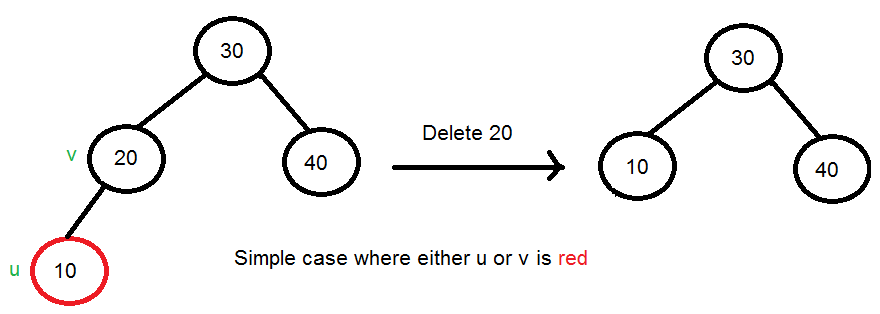3) If Both u and v are Black.
3.1) Color u as double black.  Now our task reduces to convert this double black to single black. Note that If v is leaf, then u is NULL and color of NULL is considered black. So the deletion of a black leaf also causes a double black.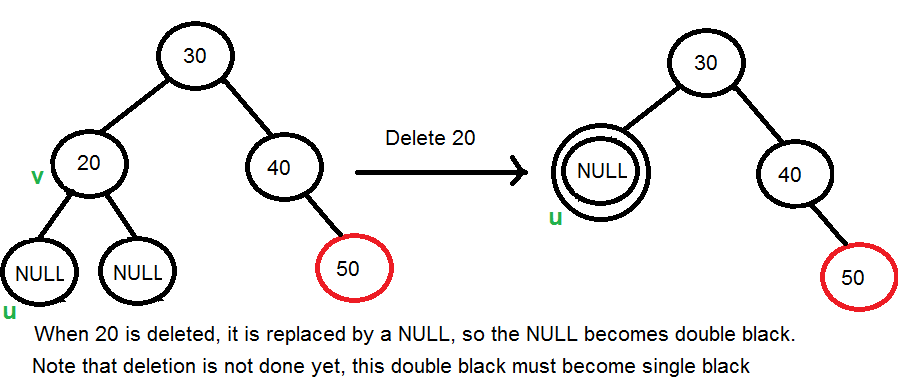3.2) Do following while the current node u is double black, and it is not the root. Let sibling of node be s
….(a): If sibling s is black and at least one of sibling’s children is red, perform rotation(s). Let the red child of s be r. This case can be divided in four subcases depending upon positions of s and r.
…………..(i) Left Left Case (s is left child of its parent and r is left child of s or both children of s are red). This is mirror of right right case shown in below diagram.
…………..(ii) Left Right Case (s is left child of its parent and r is right child). This is mirror of right left case shown in below diagram.
…………..(iii) Right Right Case (s is right child of its parent and r is right child of s or both children of s are red)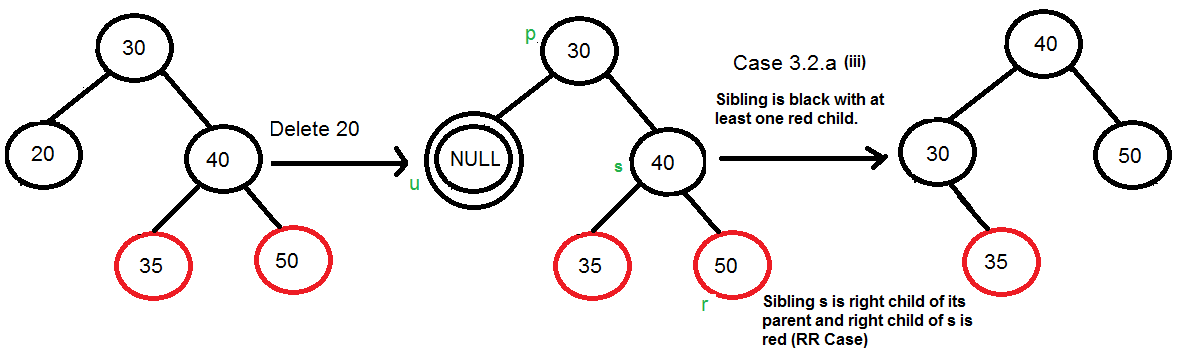…………..(iv) Right Left Case (s is right child of its parent and r is left child of s)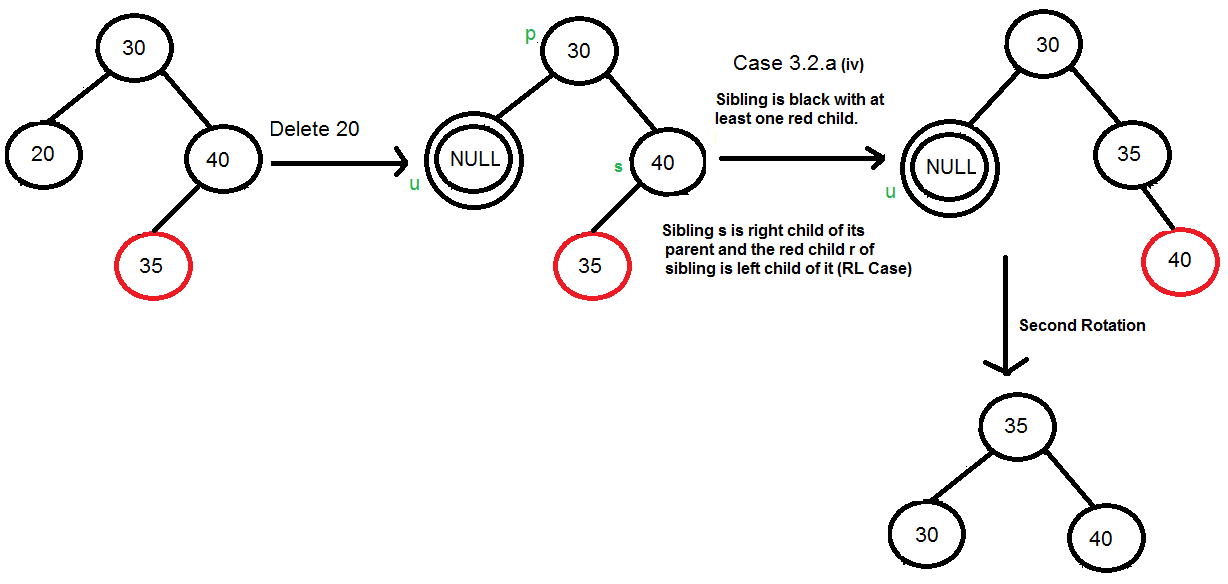…..(b): If sibling is black and its both children are black, perform recoloring, and recur for the parent if parent is black.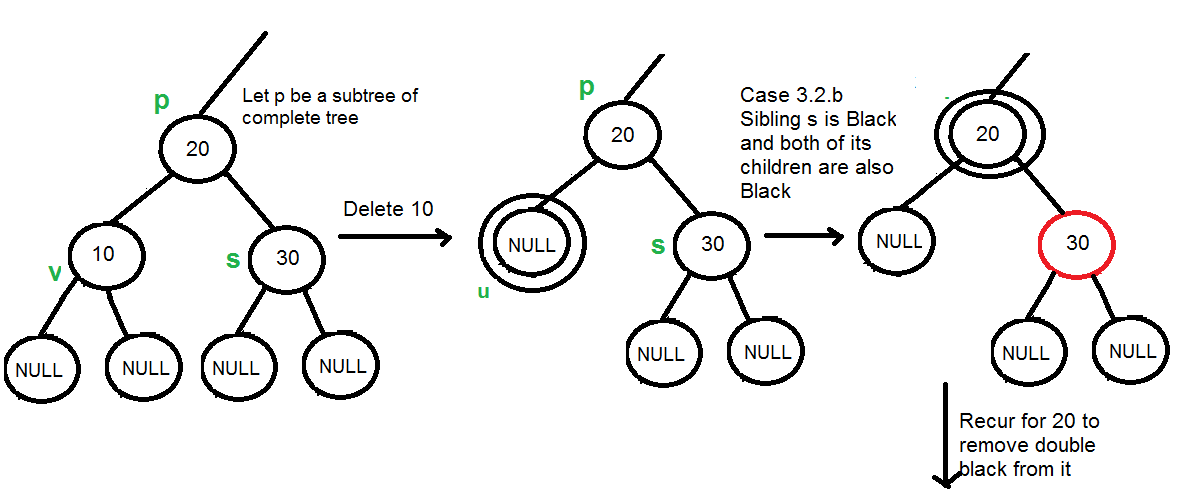In this case, if parent was red, then we didn’t need to recur for parent, we can simply make it black (red + double black = single black)
…..(c): If sibling is red, perform a rotation to move old sibling up, recolor the old sibling and parent. The new sibling is always black (See the below diagram). This mainly converts the tree to black sibling case (by rotation) and leads to case (a) or (b). This case can be divided in two subcases.
…………..(i) Left Case (s is left child of its parent). This is mirror of right right case shown in below diagram. We right rotate the parent p.
…………..(iii) Right Case (s is right child of its parent). We left rotate the parent p.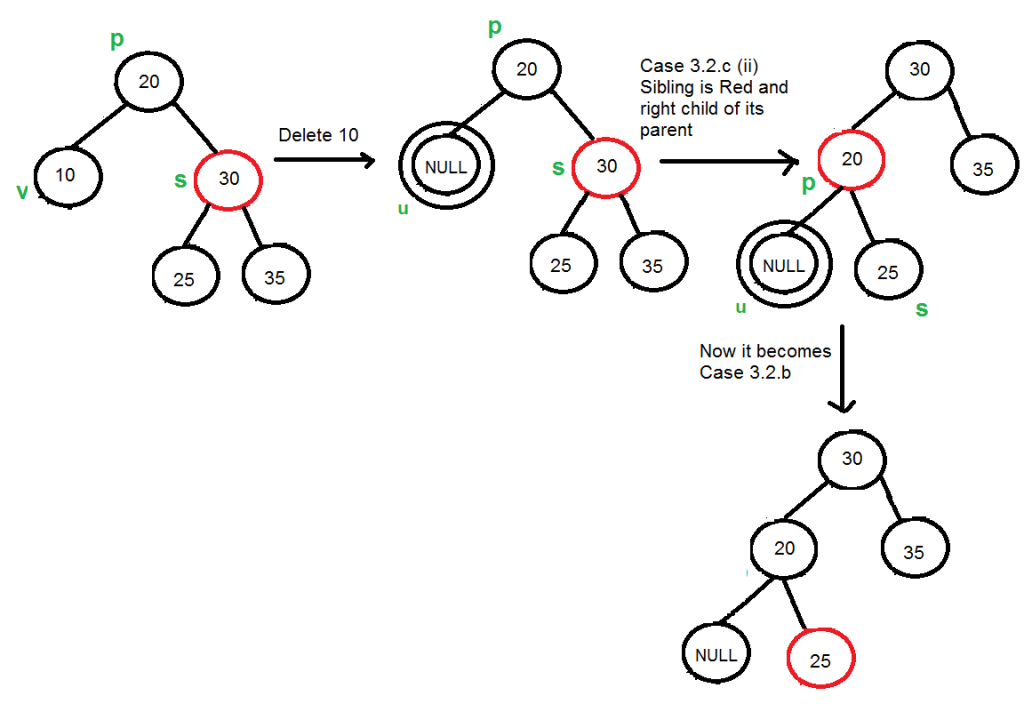3.3) If u is root, make it single black and return (Black height of complete tree reduces by 1).
below is the C++ implementation of above approach:

## CPP

 `#include ``#include ``using` `namespace` `std;` `enum` `COLOR { RED, BLACK };` `class` `Node {``public``:``  ``int` `val;``  ``COLOR color;``  ``Node *left, *right, *parent;` `  ``Node(``int` `val) : val(val) {``    ``parent = left = right = NULL;` `    ``// Node is created during insertion``    ``// Node is red at insertion``    ``color = RED;``  ``}` `  ``// returns pointer to uncle``  ``Node *uncle() {``    ``// If no parent or grandparent, then no uncle``    ``if` `(parent == NULL or parent->parent == NULL)``      ``return` `NULL;` `    ``if` `(parent->isOnLeft())``      ``// uncle on right``      ``return` `parent->parent->right;``    ``else``      ``// uncle on left``      ``return` `parent->parent->left;``  ``}` `  ``// check if node is left child of parent``  ``bool` `isOnLeft() { ``return` `this` `== parent->left; }` `  ``// returns pointer to sibling``  ``Node *sibling() {``    ``// sibling null if no parent``    ``if` `(parent == NULL)``      ``return` `NULL;` `    ``if` `(isOnLeft())``      ``return` `parent->right;` `    ``return` `parent->left;``  ``}` `  ``// moves node down and moves given node in its place``  ``void` `moveDown(Node *nParent) {``    ``if` `(parent != NULL) {``      ``if` `(isOnLeft()) {``        ``parent->left = nParent;``      ``} ``else` `{``        ``parent->right = nParent;``      ``}``    ``}``    ``nParent->parent = parent;``    ``parent = nParent;``  ``}` `  ``bool` `hasRedChild() {``    ``return` `(left != NULL and left->color == RED) or``           ``(right != NULL and right->color == RED);``  ``}``};` `class` `RBTree {``  ``Node *root;` `  ``// left rotates the given node``  ``void` `leftRotate(Node *x) {``    ``// new parent will be node's right child``    ``Node *nParent = x->right;` `    ``// update root if current node is root``    ``if` `(x == root)``      ``root = nParent;` `    ``x->moveDown(nParent);` `    ``// connect x with new parent's left element``    ``x->right = nParent->left;``    ``// connect new parent's left element with node``    ``// if it is not null``    ``if` `(nParent->left != NULL)``      ``nParent->left->parent = x;` `    ``// connect new parent with x``    ``nParent->left = x;``  ``}` `  ``void` `rightRotate(Node *x) {``    ``// new parent will be node's left child``    ``Node *nParent = x->left;` `    ``// update root if current node is root``    ``if` `(x == root)``      ``root = nParent;` `    ``x->moveDown(nParent);` `    ``// connect x with new parent's right element``    ``x->left = nParent->right;``    ``// connect new parent's right element with node``    ``// if it is not null``    ``if` `(nParent->right != NULL)``      ``nParent->right->parent = x;` `    ``// connect new parent with x``    ``nParent->right = x;``  ``}` `  ``void` `swapColors(Node *x1, Node *x2) {``    ``COLOR temp;``    ``temp = x1->color;``    ``x1->color = x2->color;``    ``x2->color = temp;``  ``}` `  ``void` `swapValues(Node *u, Node *v) {``    ``int` `temp;``    ``temp = u->val;``    ``u->val = v->val;``    ``v->val = temp;``  ``}` `  ``// fix red red at given node``  ``void` `fixRedRed(Node *x) {``    ``// if x is root color it black and return``    ``if` `(x == root) {``      ``x->color = BLACK;``      ``return``;``    ``}` `    ``// initialize parent, grandparent, uncle``    ``Node *parent = x->parent, *grandparent = parent->parent,``         ``*uncle = x->uncle();` `    ``if` `(parent->color != BLACK) {``      ``if` `(uncle != NULL && uncle->color == RED) {``        ``// uncle red, perform recoloring and recurse``        ``parent->color = BLACK;``        ``uncle->color = BLACK;``        ``grandparent->color = RED;``        ``fixRedRed(grandparent);``      ``} ``else` `{``        ``// Else perform LR, LL, RL, RR``        ``if` `(parent->isOnLeft()) {``          ``if` `(x->isOnLeft()) {``            ``// for left right``            ``swapColors(parent, grandparent);``          ``} ``else` `{``            ``leftRotate(parent);``            ``swapColors(x, grandparent);``          ``}``          ``// for left left and left right``          ``rightRotate(grandparent);``        ``} ``else` `{``          ``if` `(x->isOnLeft()) {``            ``// for right left``            ``rightRotate(parent);``            ``swapColors(x, grandparent);``          ``} ``else` `{``            ``swapColors(parent, grandparent);``          ``}` `          ``// for right right and right left``          ``leftRotate(grandparent);``        ``}``      ``}``    ``}``  ``}` `  ``// find node that do not have a left child``  ``// in the subtree of the given node``  ``Node *successor(Node *x) {``    ``Node *temp = x;` `    ``while` `(temp->left != NULL)``      ``temp = temp->left;` `    ``return` `temp;``  ``}` `  ``// find node that replaces a deleted node in BST``  ``Node *BSTreplace(Node *x) {``    ``// when node have 2 children``    ``if` `(x->left != NULL and x->right != NULL)``      ``return` `successor(x->right);` `    ``// when leaf``    ``if` `(x->left == NULL and x->right == NULL)``      ``return` `NULL;` `    ``// when single child``    ``if` `(x->left != NULL)``      ``return` `x->left;``    ``else``      ``return` `x->right;``  ``}` `  ``// deletes the given node``  ``void` `deleteNode(Node *v) {``    ``Node *u = BSTreplace(v);` `    ``// True when u and v are both black``    ``bool` `uvBlack = ((u == NULL or u->color == BLACK) and (v->color == BLACK));``    ``Node *parent = v->parent;` `    ``if` `(u == NULL) {``      ``// u is NULL therefore v is leaf``      ``if` `(v == root) {``        ``// v is root, making root null``        ``root = NULL;``      ``} ``else` `{``        ``if` `(uvBlack) {``          ``// u and v both black``          ``// v is leaf, fix double black at v``          ``fixDoubleBlack(v);``        ``} ``else` `{``          ``// u or v is red``          ``if` `(v->sibling() != NULL)``            ``// sibling is not null, make it red"``            ``v->sibling()->color = RED;``        ``}` `        ``// delete v from the tree``        ``if` `(v->isOnLeft()) {``          ``parent->left = NULL;``        ``} ``else` `{``          ``parent->right = NULL;``        ``}``      ``}``      ``delete` `v;``      ``return``;``    ``}` `    ``if` `(v->left == NULL or v->right == NULL) {``      ``// v has 1 child``      ``if` `(v == root) {``        ``// v is root, assign the value of u to v, and delete u``        ``v->val = u->val;``        ``v->left = v->right = NULL;``        ``delete` `u;``      ``} ``else` `{``        ``// Detach v from tree and move u up``        ``if` `(v->isOnLeft()) {``          ``parent->left = u;``        ``} ``else` `{``          ``parent->right = u;``        ``}``        ``delete` `v;``        ``u->parent = parent;``        ``if` `(uvBlack) {``          ``// u and v both black, fix double black at u``          ``fixDoubleBlack(u);``        ``} ``else` `{``          ``// u or v red, color u black``          ``u->color = BLACK;``        ``}``      ``}``      ``return``;``    ``}` `    ``// v has 2 children, swap values with successor and recurse``    ``swapValues(u, v);``    ``deleteNode(u);``  ``}` `  ``void` `fixDoubleBlack(Node *x) {``    ``if` `(x == root)``      ``// Reached root``      ``return``;` `    ``Node *sibling = x->sibling(), *parent = x->parent;``    ``if` `(sibling == NULL) {``      ``// No sibiling, double black pushed up``      ``fixDoubleBlack(parent);``    ``} ``else` `{``      ``if` `(sibling->color == RED) {``        ``// Sibling red``        ``parent->color = RED;``        ``sibling->color = BLACK;``        ``if` `(sibling->isOnLeft()) {``          ``// left case``          ``rightRotate(parent);``        ``} ``else` `{``          ``// right case``          ``leftRotate(parent);``        ``}``        ``fixDoubleBlack(x);``      ``} ``else` `{``        ``// Sibling black``        ``if` `(sibling->hasRedChild()) {``          ``// at least 1 red children``          ``if` `(sibling->left != NULL and sibling->left->color == RED) {``            ``if` `(sibling->isOnLeft()) {``              ``// left left``              ``sibling->left->color = sibling->color;``              ``sibling->color = parent->color;``              ``rightRotate(parent);``            ``} ``else` `{``              ``// right left``              ``sibling->left->color = parent->color;``              ``rightRotate(sibling);``              ``leftRotate(parent);``            ``}``          ``} ``else` `{``            ``if` `(sibling->isOnLeft()) {``              ``// left right``              ``sibling->right->color = parent->color;``              ``leftRotate(sibling);``              ``rightRotate(parent);``            ``} ``else` `{``              ``// right right``              ``sibling->right->color = sibling->color;``              ``sibling->color = parent->color;``              ``leftRotate(parent);``            ``}``          ``}``          ``parent->color = BLACK;``        ``} ``else` `{``          ``// 2 black children``          ``sibling->color = RED;``          ``if` `(parent->color == BLACK)``            ``fixDoubleBlack(parent);``          ``else``            ``parent->color = BLACK;``        ``}``      ``}``    ``}``  ``}` `  ``// prints level order for given node``  ``void` `levelOrder(Node *x) {``    ``if` `(x == NULL)``      ``// return if node is null``      ``return``;` `    ``// queue for level order``    ``queue q;``    ``Node *curr;` `    ``// push x``    ``q.push(x);` `    ``while` `(!q.empty()) {``      ``// while q is not empty``      ``// dequeue``      ``curr = q.front();``      ``q.pop();` `      ``// print node value``      ``cout << curr->val << ``" "``;` `      ``// push children to queue``      ``if` `(curr->left != NULL)``        ``q.push(curr->left);``      ``if` `(curr->right != NULL)``        ``q.push(curr->right);``    ``}``  ``}` `  ``// prints inorder recursively``  ``void` `inorder(Node *x) {``    ``if` `(x == NULL)``      ``return``;``    ``inorder(x->left);``    ``cout << x->val << ``" "``;``    ``inorder(x->right);``  ``}` `public``:``  ``// constructor``  ``// initialize root``  ``RBTree() { root = NULL; }` `  ``Node *getRoot() { ``return` `root; }` `  ``// searches for given value``  ``// if found returns the node (used for delete)``  ``// else returns the last node while traversing (used in insert)``  ``Node *search(``int` `n) {``    ``Node *temp = root;``    ``while` `(temp != NULL) {``      ``if` `(n < temp->val) {``        ``if` `(temp->left == NULL)``          ``break``;``        ``else``          ``temp = temp->left;``      ``} ``else` `if` `(n == temp->val) {``        ``break``;``      ``} ``else` `{``        ``if` `(temp->right == NULL)``          ``break``;``        ``else``          ``temp = temp->right;``      ``}``    ``}` `    ``return` `temp;``  ``}` `  ``// inserts the given value to tree``  ``void` `insert(``int` `n) {``    ``Node *newNode = ``new` `Node(n);``    ``if` `(root == NULL) {``      ``// when root is null``      ``// simply insert value at root``      ``newNode->color = BLACK;``      ``root = newNode;``    ``} ``else` `{``      ``Node *temp = search(n);` `      ``if` `(temp->val == n) {``        ``// return if value already exists``        ``return``;``      ``}` `      ``// if value is not found, search returns the node``      ``// where the value is to be inserted` `      ``// connect new node to correct node``      ``newNode->parent = temp;` `      ``if` `(n < temp->val)``        ``temp->left = newNode;``      ``else``        ``temp->right = newNode;` `      ``// fix red red voilaton if exists``      ``fixRedRed(newNode);``    ``}``  ``}` `  ``// utility function that deletes the node with given value``  ``void` `deleteByVal(``int` `n) {``    ``if` `(root == NULL)``      ``// Tree is empty``      ``return``;` `    ``Node *v = search(n), *u;` `    ``if` `(v->val != n) {``      ``cout << ``"No node found to delete with value:"` `<< n << endl;``      ``return``;``    ``}` `    ``deleteNode(v);``  ``}` `  ``// prints inorder of the tree``  ``void` `printInOrder() {``    ``cout << ``"Inorder: "` `<< endl;``    ``if` `(root == NULL)``      ``cout << ``"Tree is empty"` `<< endl;``    ``else``      ``inorder(root);``    ``cout << endl;``  ``}` `  ``// prints level order of the tree``  ``void` `printLevelOrder() {``    ``cout << ``"Level order: "` `<< endl;``    ``if` `(root == NULL)``      ``cout << ``"Tree is empty"` `<< endl;``    ``else``      ``levelOrder(root);``    ``cout << endl;``  ``}``};` `int` `main() {``  ``RBTree tree;` `  ``tree.insert(7);``  ``tree.insert(3);``  ``tree.insert(18);``  ``tree.insert(10);``  ``tree.insert(22);``  ``tree.insert(8);``  ``tree.insert(11);``  ``tree.insert(26);``  ``tree.insert(2);``  ``tree.insert(6);``  ``tree.insert(13);` `  ``tree.printInOrder();``  ``tree.printLevelOrder();` `  ``cout<

Output:

```Inorder:
2 3 6 7 8 10 11 13 18 22 26
Level order:
10 7 18 3 8 11 22 2 6 13 26

Deleting 18, 11, 3, 10, 22
Inorder:
2 6 7 8 13 26
Level order:
13 7 26 6 8 2 ```

References:
https://www.cs.purdue.edu/homes/ayg/CS251/slides/chap13c.pdf
Introduction to Algorithms 3rd Edition by Clifford Stein, Thomas H. Cormen, Charles E. Leiserson, Ronald L. Rivest